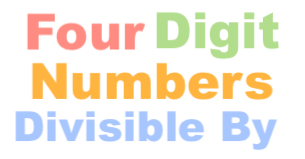Four Digit Numbers Divisible By CalculatorThe Four Digit Numbers Divisible By Calculator can calculate all the 4-digit numbers that can be divided by a number.

In other words, you have a number and you want to know all the 4-digit numbers that when divided by your number will result in a whole number.

Here are some examples of what this calculator can answer:

Four digit numbers divisible by 9

4-digit numbers divisible by 11

Four digit numbers divisible by 6

4-digit numbers divisible by 3

Four digit numbers divisible by 8

Four digit numbers divisible by 5

Four digit numbers divisible by 10

4-digit numbers divisible by 7